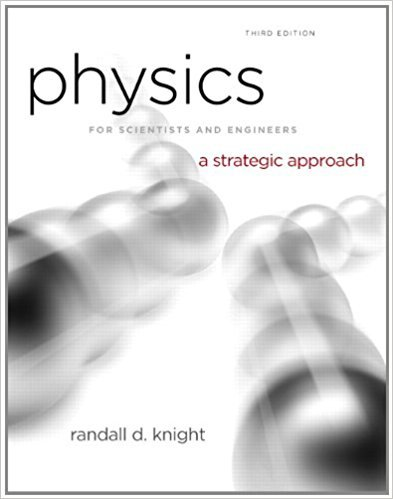×
Log in to StudySoup
Get Full Access to Physics For Scientists And Engineers: A Strategic Approach With Modern Physics - 3 Edition - Chapter 17 - Problem 77cp
Join StudySoup for FREE
Get Full Access to Physics For Scientists And Engineers: A Strategic Approach With Modern Physics - 3 Edition - Chapter 17 - Problem 77cp

Already have an account? Login here
×
Reset your password

# FIGURE shows a thermodynamic process followed by 120 mg ofISBN: 9780321740908 69

## Solution for problem 77CP Chapter 17

Physics for Scientists and Engineers: A Strategic Approach with Modern Physics | 3rd Edition

• Textbook Solutions
• 2901 Step-by-step solutions solved by professors and subject experts
• Get 24/7 help from StudySoup virtual teaching assistantsPhysics for Scientists and Engineers: A Strategic Approach with Modern Physics | 3rd Edition

4 5 1 404 Reviews
25
2
Problem 77CP

Problem 77CP

FIGURE shows a thermodynamic process followed by 120 mg of helium.

a. Determine the pressure (in atm), temperature (in °C), and volume (in cm3 ) of the gas at points 1, 2, and 3. Put your results in a table for easy reading.

b. How much work is done on the gas during each of the three segments?

c. How much heat is transferred to or from the gas during each of the three segments?

FIGUREStep-by-Step Solution:

Solution 77CP

Step 1 of 3

Part a

We are required to calculate the pressure, temperature and volume at the given points 1,2 and 3.

At the point 1, the pressure is 3 atm. The volume is 1000 cm3.

PressureatmPa

Volumecm3 = 0.001 m3

The mass of helium ismg = 120g

The molar mass of helium is 4.0 g.

Therefore, the number of moles of helium is= 30= 0.03 mole

Therefore, the temperature of helium at the point 1 is,KK0C0C

Therefore, at the point 1,

 P1 V1 T1 3.0 atm 1000 cm3 9420 C

The system reaches point 2 after an isothermal expansion.

At this point, the volume iscm3 = 0.003 m3

The temperature is 9420 C  as the process is isothermal.

The process is isothermal and the volume increases by three times. So, the pressure at this point will be reduced by three times. So, the pressure at the point 2 is 1 atm.

Therefore, at the point 2,

 P2 V2 T2 1.0 atm 3000 cm3 9420 C

The system reaches point 3 at constant volume. This is followed by an adiabatic compression to state 1. Helium has.

Therefore, to calculate the pressure the point 3, we have to consider the following equation,atmatm

Therefore, the pressure at the point 3 is 0.48 atm.

For an adiabatic process, the temperature of the point 3 can be calculated from the following equation,KKK0C0C

Therefore, the temperature at the point 3 is 3100C.

Therefore, at the point 3,

 P3 V3 T3 0.48 atm 3000 cm3 3100 C

Step 2 of 3

Step 3 of 3

##### ISBN: 9780321740908

The answer to “FIGURE shows a thermodynamic process followed by 120 mg of helium.a. Determine the pressure (in atm), temperature (in °C), and volume (in cm3 ) of the gas at points 1, 2, and 3. Put your results in a table for easy reading.________________b. How much work is done on the gas during each of the three segments?________________c. How much heat is transferred to or from the gas during each of the three segments?FIGURE” is broken down into a number of easy to follow steps, and 72 words. This full solution covers the following key subjects: gas, much, segments, during, figure. This expansive textbook survival guide covers 17 chapters, and 1439 solutions. The full step-by-step solution to problem: 77CP from chapter: 17 was answered by , our top Physics solution expert on 08/30/17, 04:34AM. Since the solution to 77CP from 17 chapter was answered, more than 234 students have viewed the full step-by-step answer. This textbook survival guide was created for the textbook: Physics for Scientists and Engineers: A Strategic Approach with Modern Physics, edition: 3. Physics for Scientists and Engineers: A Strategic Approach with Modern Physics was written by and is associated to the ISBN: 9780321740908.

Unlock Textbook Solution

Enter your email below to unlock your verified solution to:

FIGURE shows a thermodynamic process followed by 120 mg of